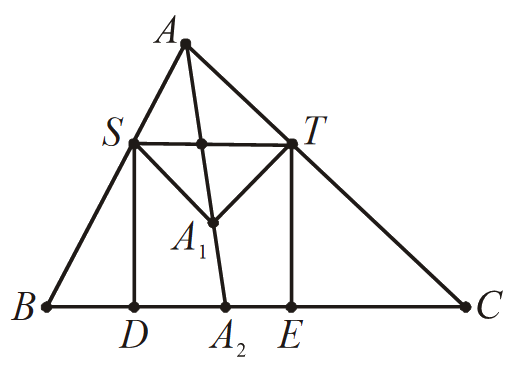# Trigonometry Set-4

Go back to  'SOLVED EXAMPLES'

Example - 9

Let $$x, y, z$$ be positive real numbers such that \begin{align}x + y + z = 1\end{align}. Determine the minimum value of

\begin{align}\frac{1}{x} + \frac{4}{y} + \frac{9}{z}\end{align}

Solution: An application of Cauchy-Schwarz inequality makes this is a one-step problem. Nevertheless, we present a proof which involves only the easier inequality \begin{align}{x^2} + {y^2} \ge \;2xy\end{align} for real numbers $$x$$ and $$y,$$ by setting first \begin{align}x = \tan b\;{\rm{and}}\;y = 2\tan b\end{align} and second \begin{align}x = \tan a\;{\rm{and}}\;y = \cot a\end{align}.

Clearly, $$z$$ is a real number in the interval [0, 1]. Hence there is an angle a such that \begin{align}z = {\sin ^2}a.\end{align} Then \begin{align}x + y = 1 - {\sin ^2}a = {\cos ^2}a,\;{\rm{or}}\;\frac{x}{{{{\cos }^2}a}} + \frac{y}{{{{\cos }^2}a}} = 1\end{align}. For an angle $$b,$$ we have \begin{align}{\cos ^2}b + {\sin ^2}b = 1\end{align}. Hence, we can set \begin{align}x = {\cos ^2}a\;{\cos ^2}b,\;y = {\cos ^2}a\;{\sin ^2}b\end{align}  for some angle $$b.$$ It suffices to find the minimum value of

$P = {\sec ^2}a{\sec ^2}b + 4{\sec ^2}a{\csc ^2}b + 9{\csc ^2}a,$

or

.$P = ({\tan ^2}a + 1)({\tan ^2}b + 1) + 4({\tan ^2}a + 1)({\cot ^2}b + 1) + 9({\cot ^2}a + 1)$

Expanding the right-hand side gives

\begin{align} P & = 14 + 5{\tan ^2}a + 9{\cot ^2}a + ({\tan ^2}b + 4{\cot ^2}b)(1 + {\tan ^2}a)\\ & > 14 + 5{\tan ^2}a + 9{\cot ^2}a + 2\tan b \cdot 2\cot b(1 + {\tan ^2}a)\\ & = 18 + 9({\tan ^2}a + {\cot ^2}a) \ge 18 + 9 \cdot 2\tan a\cot a = 36 \end{align}

Equality holds when \begin{align}\tan a = \cot a\;{\rm{and}}\;\tan b = 2\cot b\end{align}, which implies that \begin{align}{\cos ^2}a = {\sin ^2}a\;{\rm{and}}\end{align} \begin{align}{\cos ^2}b = {\sin ^2}b\end{align}. Because \begin{align}{\sin ^2}\theta + {\cos ^2}\theta = 1\end{align}, equality holds when \begin{align}{\cos ^2}a = \frac{1}{2}\;{\text{and}}\;{\cos ^2}b = \frac{1}{3};\end{align} that is \begin{align}x = \frac{1}{6},\;y = \frac{1}{3},\;z = \frac{1}{2}\end{align}.

Example - 10

Let $$A_1$$be the center of the square inscribed in acute triangle $$ABC$$ with two vertices of the square on side $$BC$$ (shown in figure). Thus one of the two remaining vertices of the square lies on side $$AB$$ and the other on segment $$AC.$$ Points $$B_1$$ and $$C_1$$ are defined in a similar way for inscribed squares with two vertices on sides $$AC$$ and $$AB,$$ respectively. Prove that lines $$AA_1, BB_1, CC_1$$are concurrent.

Solution:Let line $$AA_1$$ and segment $$BC$$ intersect at $$A_2.$$ We define $$B_2$$ and $$C_2$$ analogously. By Ceva’s theorem, it suffices to show that

\begin{align}\frac{{\sin \angle BA{A_2}}}{{\sin \angle {A_2}AC}} \cdot \frac{{\sin \angle CB{B_2}}}{{\sin \angle {B_2}BA}} \cdot \frac{{\sin \angle AC{C_2}}}{{\sin \angle {C_2}CB}} = 1.\end{align}

Let the vertices of the square be $$DETS,$$ labled as shown in figure. Applying the law of sines to triangles \begin{align}AS{A_1}\end{align} and \begin{align}AT{A_1}\end{align} gives

\begin{align}\frac{{|A{A_1}|}}{{|S{A_1}|}} = \frac{{\sin \angle AS{A_1}}}{{\sin \angle SA{A_1}}} = \frac{{\sin \angle AS{A_1}}}{{\sin \angle BA{A_2}}}\;{\rm{and}}\;\frac{{|T{A_1}|}}{{|A{A_1}|}} = \frac{{\sin \angle {A_1}AT}}{{\sin \angle AT{A_1}}} = \frac{{\sin \angle {A_2}AC}}{{\sin \angle AT{A_1}}}\end{align}

Because \begin{align}|{{A}_{1}}S|=|{{A}_{1}}T|\ \text{and}\ \angle AS{{A}_{1}}=B+45{}^\text{o}\ \text{and}\ \angle AT{{A}_{1}}=C+45{}^\text{o}\end{align}, multiplying the above identities yields

\begin{align}1=\frac{|A{{A}_{1}}|}{|S{{A}_{1}}|}\cdot \frac{|T{{A}_{1}}|}{|A{{A}_{1}}|}-\frac{\sin \angle AS{{A}_{1}}}{\sin \angle BA{{A}_{2}}}\cdot \frac{\sin \angle {{A}_{2}}AC}{\sin \angle AT{{A}_{1}}}\end{align}

implying that

\begin{align}\frac{\sin \angle BA{{A}_{2}}}{\sin \angle {{A}_{2}}AC}=\frac{\sin \angle AS{{A}_{2}}}{\sin \angle AT{{A}_{1}}}=\frac{\sin (B+45{}^\text{o})}{\sin (C+45{}^\text{o})}\end{align}

In exactly the same way, we can show that

\begin{align}\frac{\sin \angle CB{{B}_{2}}}{\sin \angle {{B}_{2}}BA}=\frac{\sin (C+45{}^\text{o})}{\sin (A+45{}^\text{o})}\ \ \text{and}\ \ \frac{\sin \angle AC{{C}_{2}}}{\sin \angle {{C}_{2}}CB}=\frac{\sin (A+45{}^\text{o})}{\sin (B+45{}^\text{o})}\end{align}

Multiplying the last three identities establishes the desired result.

Learn from the best math teachers and top your exams

• Live one on one classroom and doubt clearing
• Practice worksheets in and after class for conceptual clarity
• Personalized curriculum to keep up with school